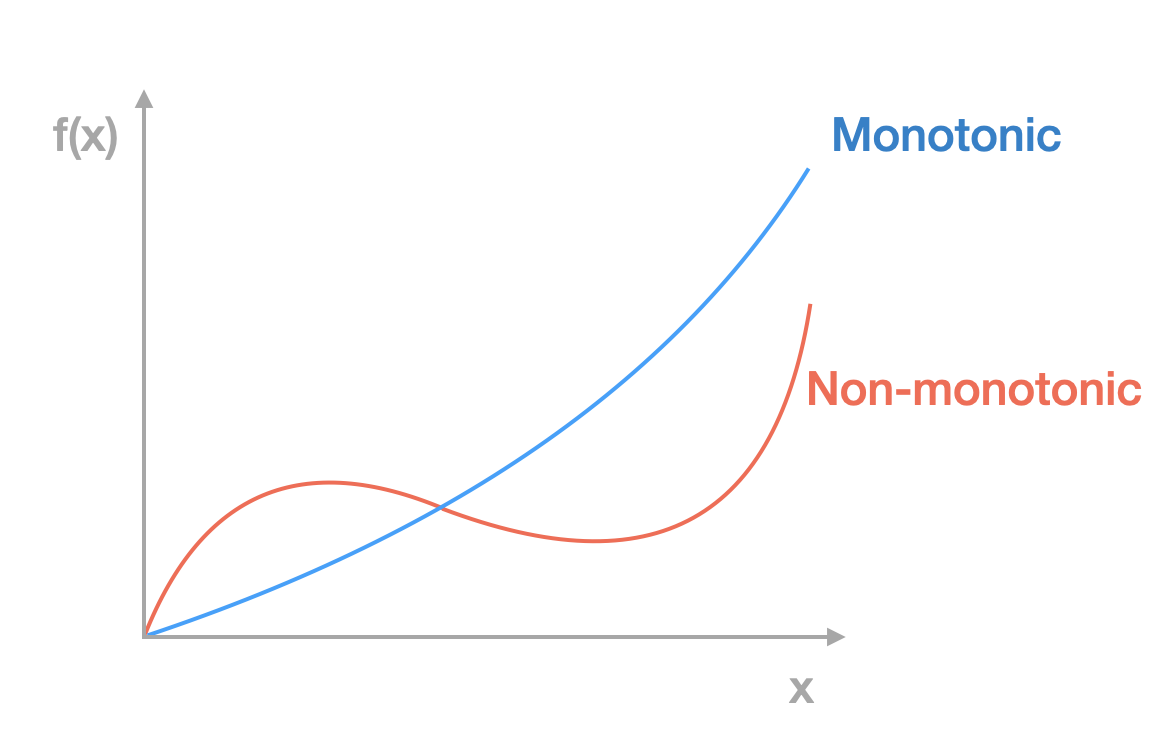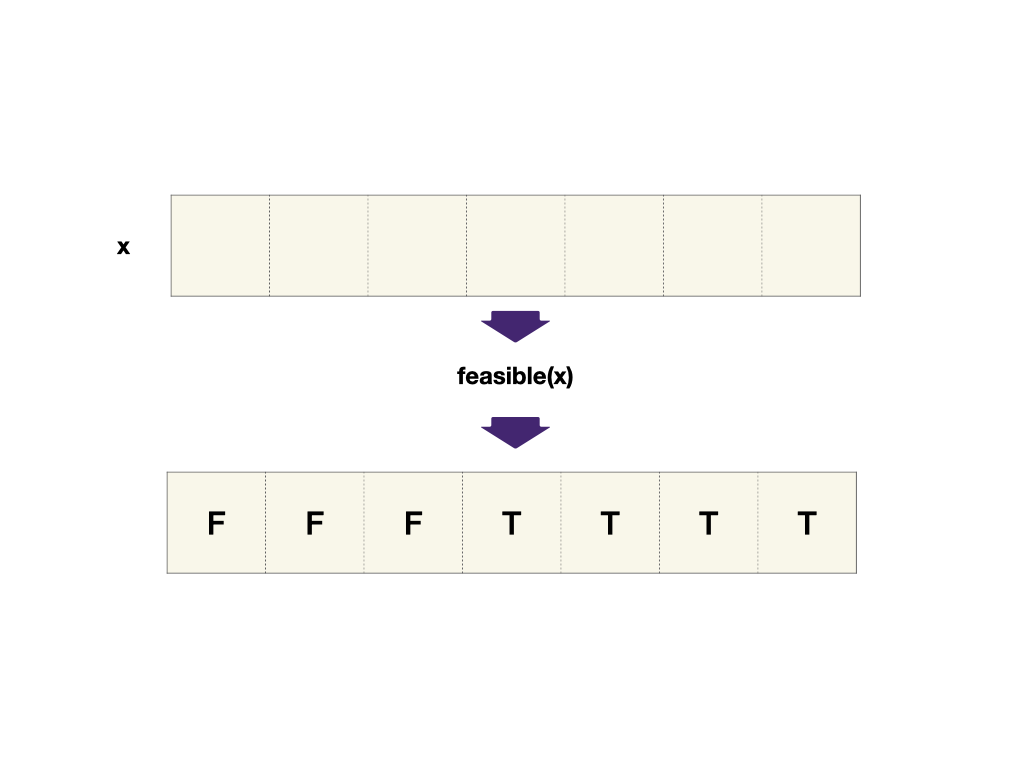# Binary Search and Monotonic Function

In the preceeding binary search problems, the arrays are always sorted. And we stated that we can use binary search whenever you make a binary decision to shrink the search range. This might sound a little abstract. In this module, we will dive a little further into when we can use binary search.

## Monotonic FunctionA monontic function is a function that is either non-decreasing or non-increasing. Given `x1` and `x2` where `x1 > x2`, we should have `f(x1) >= f(x2)`.

A sorted array is monotonic because the value increases or stays the same as the index increases.

If `f(x)` only contain boolean values `True` and `False` and think true as 1 and false as 0, then a sorted boolean array would consist of consecutive `0`s and then consecutive `1`s. For example, `FFFFTTTTT`.

## Binary Search Template

### `feasible` function

The pre-condition for binary search is to find a monotonic function `f(x)` that returns either `True` or `False`. Then the problem becomes Find the First True in a Sorted Boolean Array that we already know how to solve using binary search. We will call the function `feasible` to signify that whether the element at the current index is feasible (True) or not (False) to meet the problem constraints.``````1def binary_search(arr: List[int], target: int) -> int:
2    left, right = 0, len(arr) - 1
3    first_true_index = -1
4    while left <= right:
5        mid = (left + right) // 2
6        if feasible(mid):
7            first_true_index = mid
8            right = mid - 1
9        else:
10            left = mid + 1
11
12    return first_true_index
13``````
``````1public static int binarySearch(List<Integer> arr, int target) {
2    int left = 0;
3    int right = arr.length - 1;
4    int firstTrueIndex = -1;
5    while (left <= right) {
6        int mid = left + (right - left) / 2;
7        if (feasible(mid)) {
8            firstTrueIndex = mid;
9            right = mid - 1;
10        } else {
11            left = mid + 1;
12        }
13    }
14    return firstTrueIndex;
15}
16``````
``````1public static int BinarySearch(List<int> arr, int target)
2{
3    int left = 0;
4    int right = arr.Count - 1;
5    int firstTrueIndex = -1;
6    while (left <= right)
7    {
8        int mid = (left + right) / 2;
9        if (feasible(mid))
10        {
11            firstTrueIndex = mid;
12            right = mid - 1;
13        }
14        else
15        {
16            left = mid + 1;
17        }
18    }
19    return firstTrueIndex;
20}
21``````
``````1function binarySearch(arr, target) {
2    let left = 0;
3    let right = arr.length - 1;
4    let first_true_index = -1;
5
6    while (left <= right) {
7        let mid = Math.floor((left + right) / 2);
8        if (feasible(mid)) {
9            first_true_index = mid;
10            right = mid - 1;
11        } else {
12            left = mid + 1;
13        }
14    }
15    return first_true_index;
16}
17``````
``````1int binary_search(std::vector<int> arr, int target) {
2    int left = 0;
3    int right = arr.size() - 1;
4    int firstTrueIndex = -1;
5    while (left <= right) {
6        int mid = left + (right - left) / 2;
7        if (feasible(mid)) {
8            firstTrueIndex = mid;
9            right = mid - 1;
10        } else {
11            left = mid + 1;
12        }
13    }
14    return firstTrueIndex;
15}
16``````
``````1func binarySearch(arr []int, target int) int {
2    left := 0
3    right := len(arr) - 1
4    firstTrueIndex := -1
5    for left <= right {
6        mid := (left + right) / 2
7        if feasible(mid) {
8            firstTrueIndex = mid
9            right = mid - 1
10        } else {
11            left = mid + 1
12        }
13    }
14    return firstTrueIndex
15}
16``````
``````1(define (binary-search arr target)
2  (let search ([l 0]
3               [r (sub1 (vector-length arr))])
4    (if (<= l r)
5        (let* ([m (quotient (+ l r) 2)]
6               [v (vector-ref arr m)])
7          (cond
8            [(= v target) m]
9            [(< v target) (search (add1 m) r)]
10            [else (search l (sub1 r))]))
11        -1)))
12``````
``````1binarySearch :: Array Int Int -> Int -> Int
2binarySearch arr target = uncurry search \$ bounds arr where
3  search l r
4    | l <= r = case compare (arr A.! m) target of
5        EQ -> m
6        LT -> search (succ m) r
7        GT -> search l (pred m)
8    | otherwise = -1
9    where m = l + (r - l) `div` 2
10``````

Now the problem has become finding the `feasible` function and then mechanically applying the template.

In the Find the First True in a Sorted Boolean Array problem, the `feasibile` function is simply `arr[mid] is True`. It's tricker to find the `feasible` function in other problems. Let's look at a few examples.

Still not clear? Submit the part you don't understand to our editors.